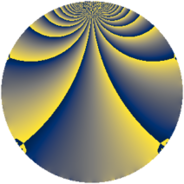# Properties

 Label 1183.1.tLevel $1183$ Weight $1$ Character orbit 1183.t Rep. character $\chi_{1183}(699,\cdot)$ Character field $\Q(\zeta_{6})$ Dimension $12$ Newform subspaces $1$ Sturm bound $121$ Trace bound $0$

# Related objects

## Defining parameters

 Level: $$N$$ $$=$$ $$1183 = 7 \cdot 13^{2}$$ Weight: $$k$$ $$=$$ $$1$$ Character orbit: $$[\chi]$$ $$=$$ 1183.t (of order $$6$$ and degree $$2$$) Character conductor: $$\operatorname{cond}(\chi)$$ $$=$$ $$91$$ Character field: $$\Q(\zeta_{6})$$ Newform subspaces: $$1$$ Sturm bound: $$121$$ Trace bound: $$0$$

## Dimensions

The following table gives the dimensions of various subspaces of $$M_{1}(1183, [\chi])$$.

Total New Old
Modular forms 40 32 8
Cusp forms 12 12 0
Eisenstein series 28 20 8

The following table gives the dimensions of subspaces with specified projective image type.

$$D_n$$ $$A_4$$ $$S_4$$ $$A_5$$
Dimension 12 0 0 0

## Trace form

 $$12q + 4q^{4} - 6q^{9} + O(q^{10})$$ $$12q + 4q^{4} - 6q^{9} - 4q^{14} - 2q^{16} + 4q^{22} - 2q^{23} - 12q^{25} + 2q^{29} + 4q^{36} - 2q^{43} + 6q^{49} - 4q^{53} - 4q^{56} + 4q^{74} + 4q^{77} - 4q^{79} - 6q^{81} - 8q^{88} - 12q^{92} + O(q^{100})$$

## Decomposition of $$S_{1}^{\mathrm{new}}(1183, [\chi])$$ into newform subspaces

Label Dim. $$A$$ Field Image CM RM Traces $q$-expansion
$$a_2$$ $$a_3$$ $$a_5$$ $$a_7$$
1183.1.t.a $$12$$ $$0.590$$ 12.0.$$\cdots$$.1 $$D_{7}$$ $$\Q(\sqrt{-7})$$ None $$0$$ $$0$$ $$0$$ $$0$$ $$q+(\beta _{1}+\beta _{2}-\beta _{6})q^{2}+(-1+\beta _{3}+\beta _{5}+\cdots)q^{4}+\cdots$$